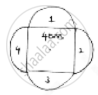# A Square Water Tank Has Its Side Equal to 40m, There Are 4 Semicircular Flower Beds Grassy Plots All Around It. Find the Cost of Turfing the Plot at Rs 1.25/Sq.M - Mathematics

Sum

A square water tank has its side equal to 40m, there are 4 semicircular flower beds grassy plots all around it. Find the cost of turfing the plot at Rs 1.25/sq.m

#### SolutionSide of water tank = 40m

Grassy plot is semicircular with radius =(side)/2=40/2= 20𝑚 = 𝑟

Area of grassy plot = 4(area od semicircular grassy plot with radius 20m)

= 4 [1/2(𝑎𝑟𝑒𝑎 𝑜𝑓 𝑐𝑖𝑟𝑐𝑙𝑒 𝑤𝑖𝑡ℎ 𝑟𝑎𝑑𝑖𝑢𝑠)]

= 4 ×1/2× pi(20)^2

= 2 × 20 × 20 × 𝜋 = 800𝜋 𝑚2.

Cost of turfing 1m2 = Rs. 1.25

Total cost of turfing the grassy plot around tank

= 800𝜋 × 1.25

= 1000𝜋

= 1000 × 3.14

= Rs. 3140

Concept: Circumference of a Circle
Is there an error in this question or solution?